# The Linking Homomorphism of One-dimensional Minimal Sets

Alex Clark and Michael C. Sullivan

 Abstract: We introduce a way of characterizing the linking of one-dimensional minimal sets in three-dimensional flows and carry out the characterization for some minimal sets within flows modeled by templates.

## Minimal Sets

If f is a continuous flow or map on a space X, then a closed subset M of X is a minimal set of f if M is invariant under f but contains no proper, non-empty, closed set which is also invariant. Periodic orbits are minmal sets, but more complicated minimal sets exist.

References:

• Qualitative Theory of Differential Equations, Nemytskii & Stepanov, Princeton University Press, 1960.
• Lectures on Topological Dynamics, Robert Ellis, Benjamin, Inc., 1969.

## Sturmian Minimal Sets

For each irrational number a in the open interval (0,1), a Strumian minimal set can be generated by the following algorythm. Let t be a real number. Let bn = t+ na mod 1. Then define xn to be 0 when bn is less than or equal to a or 1 otherwise. Then (xn)n>0 is a (one-sided) Sturmian minimal set. Sturmian minimal sets have "minimal block growth." Thus, they are the simplest minimal sets after periodic orbits.

References:

• Gottschalk, W. H., Hedlund, G., Topological dynamics, A. M. S. Colloquium Publications, Vol. 36., 1955.
• Coven, E. M.; Hedlund, G. A., Sequences with minimal block growth, Math. Systems Theory 7 (1973), 138--153.
• Paul, M. E., Minimal symbolic flows having minimal block growth, Math. Systems Theory 8 (1974/75), no. 4, 309--315.
Below is a maple procedure for generating Sturmian minimal sets:

> sturmian:=proc(alpha, t, numterms)
local i, word;
word:=[];
for i from 1 to numterms do
if (evalf(frac(t+i*alpha)) <= evalf(alpha)) then word:=[op(word),0] else word:=[op(word),1] fi;
od;
word;
end:

> sturmian(sqrt(1/2),0, 100);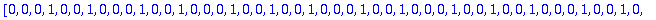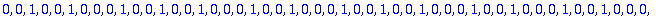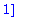## Lorenz Template

The Lorenz template is a branched 2-manifold with a semi-flow that gives a geometic model of the famous Lorenz attractor. Orbits in the Lorenz template are associated to the full 2-shift, and so contain Sturmian minimal sets.

Refernces:

• Birman, J. S.; Williams, R. F., Knotted periodic orbits in dynamical systems. I. Lorenz's equations. Topology 22 (1983), no. 1, 47--82.
• Birman, J. S.; Williams, R. F., Knotted periodic orbits in dynamical system. II. Knot holders for fibered knots, Contemp. Math., 20, Amer. Math. Soc., (1983), 1--60.
• Ghrist, R.; Holmes, P. J.; Sullivan, M. C., Knots and links in three-dimensional flows. Lecture Notes in Mathematics, 1654 (1997), Springer-Verlag, Berlin.
We have used a Maple procedure to plot the Sturmian minimal set above in the Lorenz template.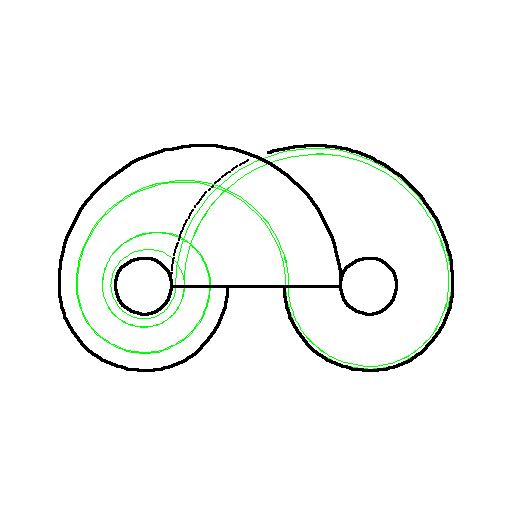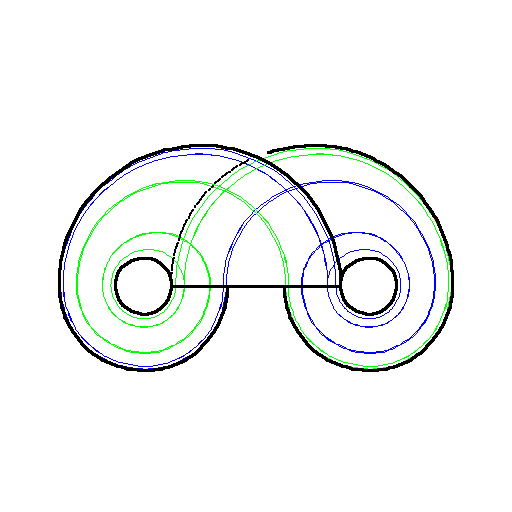Careful inspection gives a preliminary linking matrix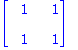, whose Smith normal form is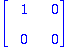.